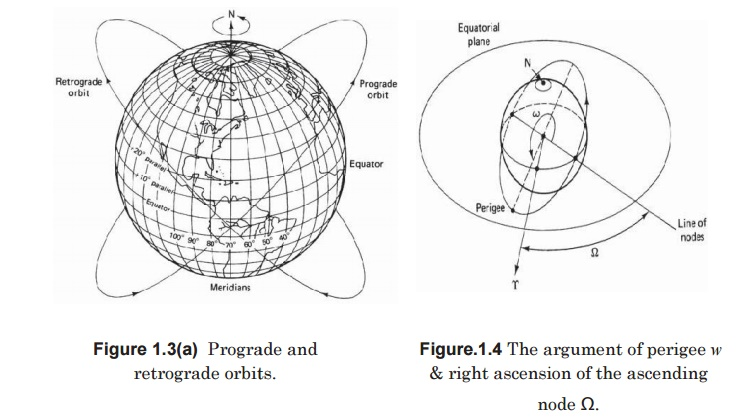Home | | Satellite Communication | Orbital Parameters

# Orbital Parameters

Apogee: A point for a satellite farthest from the Earth. It is denoted as ha.

orbital parameters:

Apogee: A point for a satellite farthest from the Earth. It is denoted as ha.

Perigee: A point for a satellite closest from the Earth. It is denoted as hp.

Line of Apsides: Line joining perigee and apogee through centre of the Earth.

It is the major axis of the orbit. One-half of this lineâ€źs length is the semi-major axis equivalents to satelliteâ€źs mean distance from the Earth.

Ascending Node: The point where the orbit crosses the equatorial plane going from north to south.

Descending Node: The point where the orbit crosses the equatorial plane going from south to north.

Inclination: the angle between the orbital plane and the Earthâ€źs equatorial plane. Its measured at the ascending node from the equator to the orbit, going from East to North. Also, this angle is commonly denoted as i.

Line of Nodes: the line joining the ascending and descending nodes through the centre of Earth.

Prograde Orbit: an orbit in which satellite moves in the same direction as the Earthâ€źs rotation. Its inclination is always between 00 to 900. Many satellites follow this path as Earthâ€źs velocity makes it easier to lunch these satellites.

Retrograde Orbit: an orbit in which satellite moves in the same direction counter to the Earthâ€źs rotation.

Argument of Perigee: An angle from the point of perigee measure in the orbital plane at the Earthâ€źs centre, in the direction of the satellite motion.

Right ascension of ascending node: The definition of an orbit in space, the position of ascending node is specified. But as the Earth spins, the longitude of ascending node changes and cannot be used for reference. Thus for practical determination of an orbit, the longitude and time of crossing the ascending node is used.For absolute measurement, a fixed reference point in space is required.

It could also be defined as â€śright ascension of the ascending node; right ascension is the angular position measured eastward along the celestial equator from the vernal equinox vector to the hour circle of the objectâ€ť.

Mean anamoly: It gives the average value to the angular position of the satellite with reference to the perigee.

True anamoly: It is the angle from point of perigee to the satelliteâ€źs position, measure at the Earthâ€źs centre.Study Material, Lecturing Notes, Assignment, Reference, Wiki description explanation, brief detail
Satellite Communication : Satellite Orbits : Orbital Parameters |